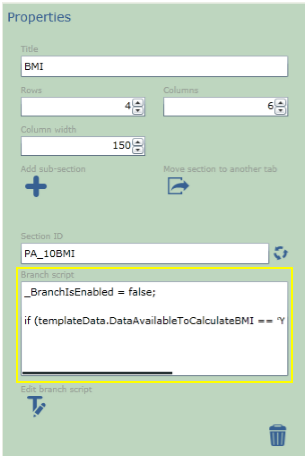# Background

In a template there may be questions or sections that you want answered only if a previous question is completed with a specific answer. You can do this using a little bit of java-script on the question or section that you want to be conditional. In the following examples we are going to demonstrate how to do this.

# Terminology

To allow us to describe how conditional logic works it would be helpful if we used the same words. Here is an explanation of the terminology we will be using;

 Terminology Description Parent Question Conditional logic allows you to hide or show questions based on the answer of a parent question.Child Question The questions that we are hiding or showing are called child questions. templateData This refers to all of the data in the template, you cannot rename this. Column_id The unique identifier given to each question. This value can be found in the property window of the question. When you select a question, the property bar for the question is shown on the right hand side. The column_id is in here.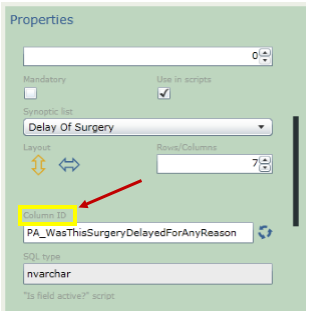_IsFieldActive == (true or false) This is how you tell the question to be enabled (true) or not (false). _BranchIsEnabled== (true or false) This is how you tell the section to be enabled (true) or not (false). == Is a comparison operator which means equal to. != Is a comparison operator which means not equal to. || Logic operator which means ‘or’. && Logic operator which means ‘and’. List Item Value In the property window for a list item is a property called Value,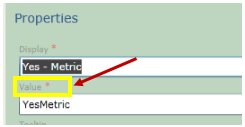# How To Make a Question Conditional based on a Drop Down List or Radio Box  Question

For this explanation we are going to use a basic example of trying to enable a label if a value from another question is selected. For the example we are going to use the question ‘Data available to calculate BMI?’.If they choose the option ‘Yes – Metric’ then we want to enable a label that says ‘BMI (Metric)’.

The properties for the parent question are,The child question properties are,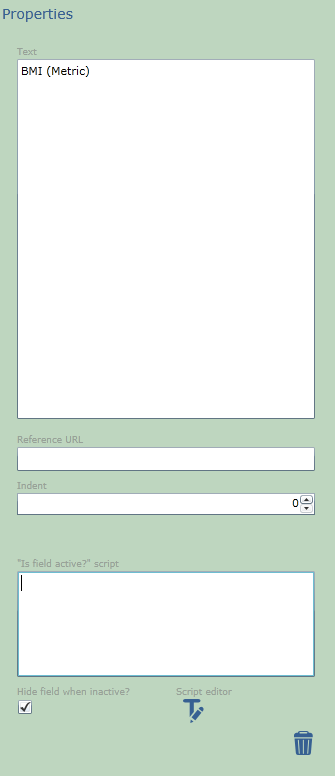To create the java-script you need to know the following:

The column id of the parent question that you want to base the conditioning on.Check off ‘Use in Scripts’ on the parent question(s) to be referenced.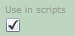The value of the item, that we want to enable the label for (this needs to be found in the Lists).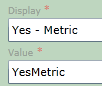# How To Make a Question Conditional based on a Check Box List

Making a child question conditional based on a parent question that uses a check box list is almost the same as the steps for a parent that uses a radio box or a drop down box. The different is in the ‘if’ statement. For a radio box the if statement looks like this,

if (templateData.DataAvailableToCalculateBMI == ‘YesMetric’) _IsFieldActive = true;

If we changed our example question to be a check box question, it would look like this,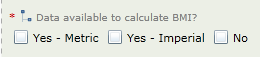So our ‘if’ statement would now look like,

if (templateData.DataAvailableToCalculateBMI.YesMetric == true) _IsFieldActive = true;

This is because when a question is a check box type question each possible answer becomes its own question.

# How To Make a Section Conditional

Making a section conditional is very similar as making a question conditional. Here the difference is the value that you set to true or false. Here is an example of the syntax.

_BranchIsEnabled = false;

if (templateData.DataAvailableToCalculateBMI == ‘YesMetric’) _BranchIsEnabled = true;

Notice instead of saying _isFieldActive it is now saying _BrachIsEnabled, otherwise the rest of the syntax is the same. This syntax needs to be placed in the Branch script editor in the properties window of the section.